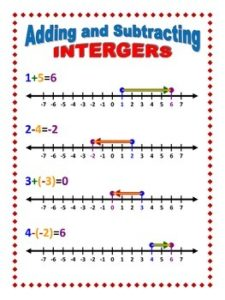# Middle School Math – Week of Sept 12-16, 2022

Welcome to a new year of learning!  We are excited about all the great things that will be happening in math this year!  We look forward to many moments of pure joy as the students discover their math prowess.This is the first of many weekly posts to tell you about what your student will be doing in math.  They will be posted at the start of the week, giving the plans for the week and various “helps” for parents and students.

Remember that you can always reach out to Mrs. VonFeldt and Mrs. Evans if you have any questions about math.

6th Grade Math – Mrs VonFeldt and Mrs Evans

Last week in 6th grade math, our students reviewed how to do multi-digit multiplication and prime factorization.  This week in 6th grade, we will be learning how to find the greatest common factor (GCF) and the least common multiple (LCM), both of which are useful in solving problems.  The LCM is often used to find common denominators for fractions that we are adding or subtracting.  The GCF is often used to find the factor that we can use to change an addition problem into a multiplication problem.  We will be using the distribution property to do this.

Here is a video that covers how to find LCM and GCF:

7th Grade Math – Mrs Evans

Last week in

to work with negative integers.  We will continue to learn how to add and subtract integers that are both negative and positive.  This is a very important skill in algebra so we will be developing a foundational skill!   We will be learning to model these problems on number lines and with counters.  We will also be using the absolute value to find answers.

Here is a great visual to help adding and subtracting integers:You can also get help with these skills by watching this video:

8th Grade Math – Mrs Evans

Last week in 8th grade math we began a review of rational numbers.  Rational numbers include fractions, mixed numbers, and decimals.  We reminded ourselves how to write these numbers in different ways.  We can turn any one of them into another.  This week we will be reviewing multiplication and division of rational numbers as well as adding and subtracting fractions with different denominators.  It is great to have time to remind ourselves of these important skills so that we can work with them effectively.

Algebra – Mrs VonFeldt

Last week the students reviewed variables and used the order of operations to evaluate expressions. This week, they are working in groups to generalize a tile pattern and write an expression that will find the number of tiles in any figure of that pattern. Students will create a poster to communicate their process.Communication can often be one of the hardest parts of solving problems, yet also one of the most important. Part of the communication process is SHOWING WORK. Please remind your student that it is always expected to show work on homework and tests.